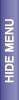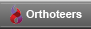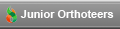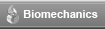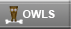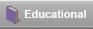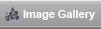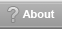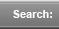# Statistics Glossary

from Prophet

> alternative hypothesis:
The null hypothesis for a statistical test is the assumption that the test uses for calculating the probability of observing a result at least as extreme as the one that occurs in the data at hand. An alternative hypothesis is one that specifies that the null hypothesis is not true.

For the one-sample t test , the null hypothesis is that the population mean equals a specific value. For a two-sided test, the alternative hypothesis is that the mean does not equal that value. It is also possible to have a one-sided test with the alternative hypothesis that the mean is greater than the specified value, if it is theoretically impossible for the mean to be less than the specified value. One could alternatively perform one-sided test with the alternative hypothesis that the mean is less than the specified value, if it were theoretically impossible for the mean to be greater than the specified value.

One-sided tests usually have more power than two-sided tests, but they require more stringent assumptions. They should only be used when those assumptions (such as the mean always being at least as large as they specified value for the one-sample t test) apply.

> between effects:
In a repeated measures ANOVA, there will be at least one factor that is measured at each level for every subject. This is a within (repeated measures) factor. For example, in an experiment in which each subject performs the same task twice, trial (or trial number) is a within factor. There may also be one or more factors that are measured at only one level for each subject, such as gender. This type of factor is a between or grouping factor.

> bias:
An estimator for a parameter is unbiased if its expected value is the true value of the parameter. Otherwise, the estimator is biased .

> binary variable:
A binary random variable is a discrete random variable that has only two possible values, such as whether a subject dies (event) or lives (non-event). Such events are often described as success vs failure.

> boxplot:A boxplot is a graph summarizing the distribution of a set of data values. The upper and lower ends of of the center box indicate the 75th and 25th percentiles of the data, the center box indicates the median, and the center + indicates the mean. Suspected outliers appear in a boxplot as individual points o or x outside the box. The o outlier values are known as outside values, and the x outlier values as far outside values.

If the difference (distance) between the 75th and 25th percentiles of the data is H , then the outside values are those values that are more than 1.5H but no more than 3H above the upper quartile, and those values that are more than 1.5H but no more than 3H below the lower quartile. The far outside values are values that are at least 3H above the upper quartile or 3H below the lower quartile.

Examples of these plots illustrate various situations.

This is a preview of the site content. To view the full text for this site, you need to log in.
If you are having problems logging in, please refer to the login help page.

 © 2005-2007 Orthoteers.co.uk - last updated by Len Funk on 06 April 2005 Medical Merketing and SEO by Blue Medical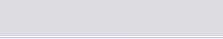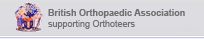Orthoteers is a non-profit educational resource. Click here for more details